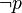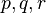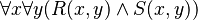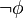Difference between revisions of "User:IssaRice/Computability and logic/List of possibilities for completeness and decidability"

Decidable logic? Complete logic? (semantic completeness) Decidable theory? Complete theory? (negation-completeness) Example or proof of non-existence
Yes Yes Yes Yes Empty theory (theory with no non-logical axioms) inside propositional logic
Yes Yes Yes No Smith's example of$T_1$ with just$\neg p$ as an axiom inside a propositional logic with propositional atoms$p,q,r$.
Yes Yes No Yes
Yes Yes No No
Yes No Yes Yes
Yes No Yes No
Yes No No Yes
Yes No No No
No Yes Yes Yes First-order logic with two binary predicates$R$ and$S$ with the axiom$\forall x \forall y (R(x,y)\wedge S(x,y))$.
No Yes Yes No
No Yes No Yes Assuming the theory is effectively axiomatized and consistent, this is not possible. To see why, suppose the theory is negation-complete. We could then computably enumerate all the theorems (since the theory is effectively axiomatized), and after a finite amount of time we will find exactly one of$\phi$ or$\neg \phi$, which gives us an algorithm for deciding whether or not$\phi$ is a theorem.
No Yes No No The theory of Robinson arithmetic inside first-order logic
No No Yes Yes
No No Yes No
No No No Yes
No No No No